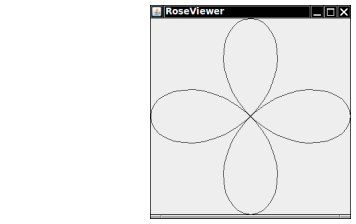Draw a picture of the “four-leaved rose” whose equation in polar coordinates is r = cos(2θ). Let θ go from 0 to 2π in 100 steps. Each time, compute r and then compute the (x, y) coordinates from the polar coordinates by using the formula

x = r · cos (θ) · y = r · sin (θ)

Here is a sample program output:Complete the following file:

### Rose.java

Use the following files:

RoseComponent.java

```import javax.swing.JComponent;
import java.awt.Graphics;
import java.awt.Graphics2D;

public class RoseComponent extends JComponent
{
public void paintComponent(Graphics g)
{
Graphics2D g2 = (Graphics2D) g;

double width = getWidth();
double height = getHeight();

Rose myRose = new Rose(width, height);
myRose.draw(g2);
}
}
```

RoseViewer.java

```import javax.swing.*;

/**
Test class driver for Rose class.
*/
public class RoseViewer
{
public static void main(String[] args)
{
JFrame frame = new JFrame();

final int FRAME_WIDTH = 300;
final int FRAME_HEIGHT = 320;

frame.setSize(FRAME_WIDTH, FRAME_HEIGHT);
frame.setTitle("RoseViewer");
frame.setDefaultCloseOperation(JFrame.EXIT_ON_CLOSE);

RoseComponent component = new RoseComponent();